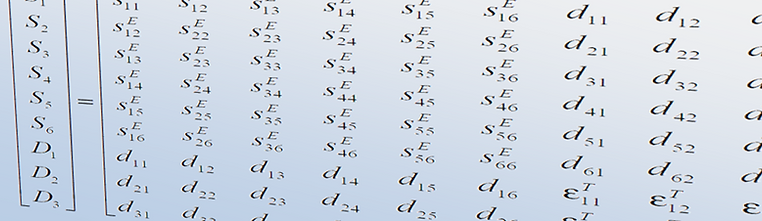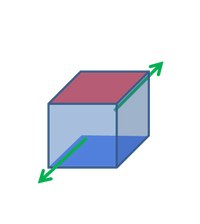The Piezoelectric Effect

# Piezoelectric Constants

## The Piezoelectric Charge Constant

So far we have looked at qualities rather than quantities of the piezo effect. In order to make also a quantitative review we need numbers, for example to compare materials or to predict a sensitivity of an accelerometer.

The number that tells us how well the material converts a mechanical force into an electrical output is called piezoelectric constant.

Most important for our applications is the piezoelectric charge constant d .

It is equal to the generated charge divided by the applied force

[d] = pC / N   (pico Coulombs per Newton)

Q is the electrical charge                         [Q] = pC     (pico Coulombs)

F  is the mechanical force                       [F] = N       (Newton)

d = Q / F      the dimension of is

However to be strictly correct this simple equation is only correct when no other influencing factor is present. That means for example that the temperature remains constant and only one single force is acting on the piezo.

## Direction Subscripts

• The directions of x, y and z of the force and the electric field are represented by 1, 2 and 3.

• The shear stress which appears about the axes 1, 2 and 3 is represented by the numbers 4, 5 and 6 respective.
A shear condition exists when we apply two opposite forces which would tend to rotate the body in the sense as indicated by the numbers 4, 5 and 6.The subscripts i and k mean:

Notation of axes

i k

dk = direction of mechanical action

i = direction of the electric field

(= direction perpendicular to the electrodes)

### Examples of Subscripts

Compression Mode.

d33 indicates the electric charge [pC] that is generated in the 3-direction (electrodes perpendicular to axis 3)

per Newton [N] force applied to the piezoelectric body also in the 3-direction.(      hover with the mouse over the picture to apply a force)Transverse Mode

d31  is the electric charge [pC] generated on electrodes perpendicular to axis 3

per unit of force (Newton) applied in the 1-direction.Shear Mode

d15   means the electric charge is developed in the 1-direction (electrodes perpendicular to axis 1)

per unit shear stress 5 applied

(5 = shear around the 2-direction, i.e. applying a force downwards at the front of the part while retaining it on the back side)In the literature or material data sheets you will find d usually with two subscripts dik

The subscripts i and k tell us in which direction i of the piezo crystal the electric charge appears while we apply a force in the direction k ( i maybe different from k )

To identify directions in a piezoelectric element, three orthogonal axes are used. These axes are named 1, 2, and 3, instead of the classical set of axes x, y, and z.

## Sensitivity of a Piezo Element

Normally when we use a piezo electric constant it is the sensitivity of a piezo element that we really want. I.e. the electric charge Q  that we obtain when we apply a certain force F.

We remember the charge constant is equal to the produced charge divided by the applied force

d = Q / F     or     Q = d·F    ( just need the adequate dik )

We know the charge is distributed over the surface, so this could lead to the feeling that increasing the surface would also increase the charge. However if we do this the force is also distributed over a larger area and the stress per surface becomes smaller in the same proportion.

This means that the output charge is independent of the size of the piezo element.